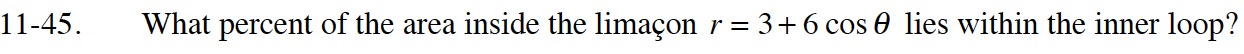### Home > CALC > Chapter 11 > Lesson 11.2.1 > Problem11-45

11-45.

What percent of the area inside the limaçon $r = 3 + 6\operatorname{cos} θ$ lies within the inner loop?Determine where the graph intersects the pole (origin):

The full area is:

$2\int_0^{\frac{2\pi}{3}}\frac{1}{2}(3+6\cos(\theta))^2d\theta$

The area of the inner loop is:

Determine the percent of the area that is inside the limacon.